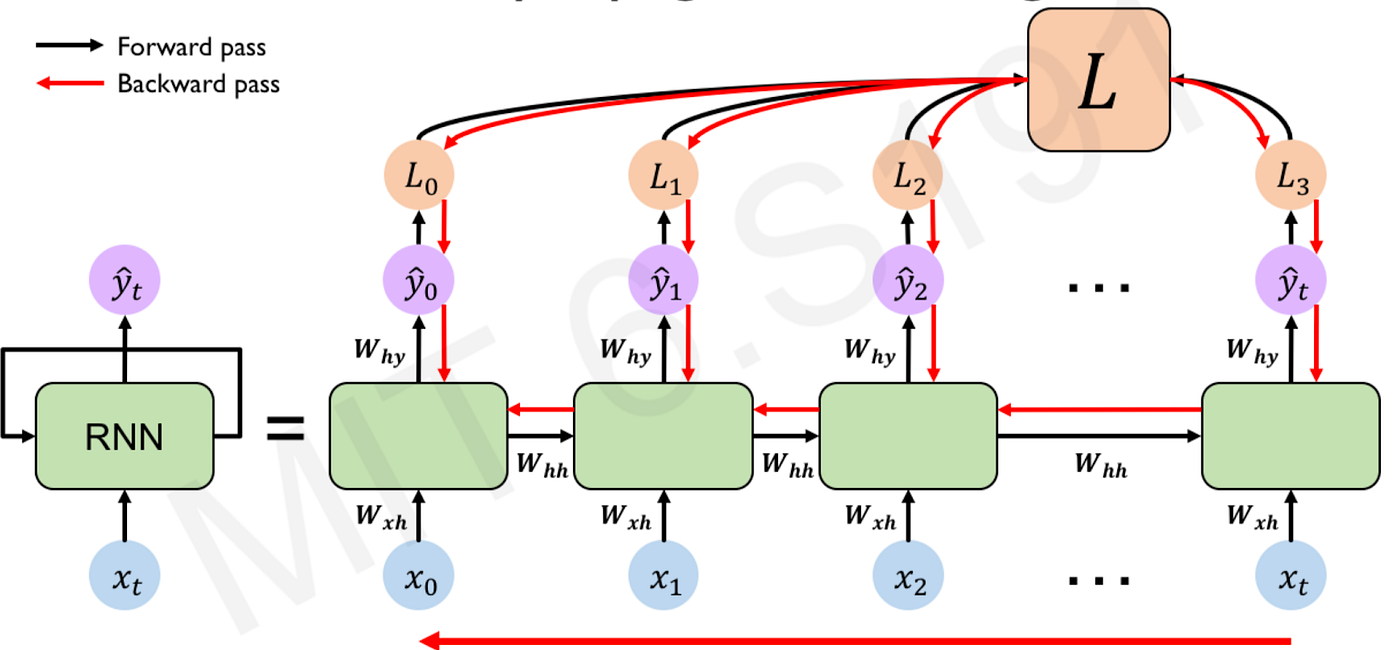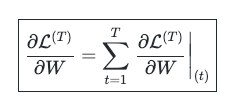1594460183

# Pytorch RNN Example (Recurrent Neural Network)

In this video we go through how to code a simple rnn, gru and lstm example. Focus is on the architecture itself rather than the data etc. and we use the simple MNIST dataset for this example.

#pytorch #rnn #neural network

## Buddha Community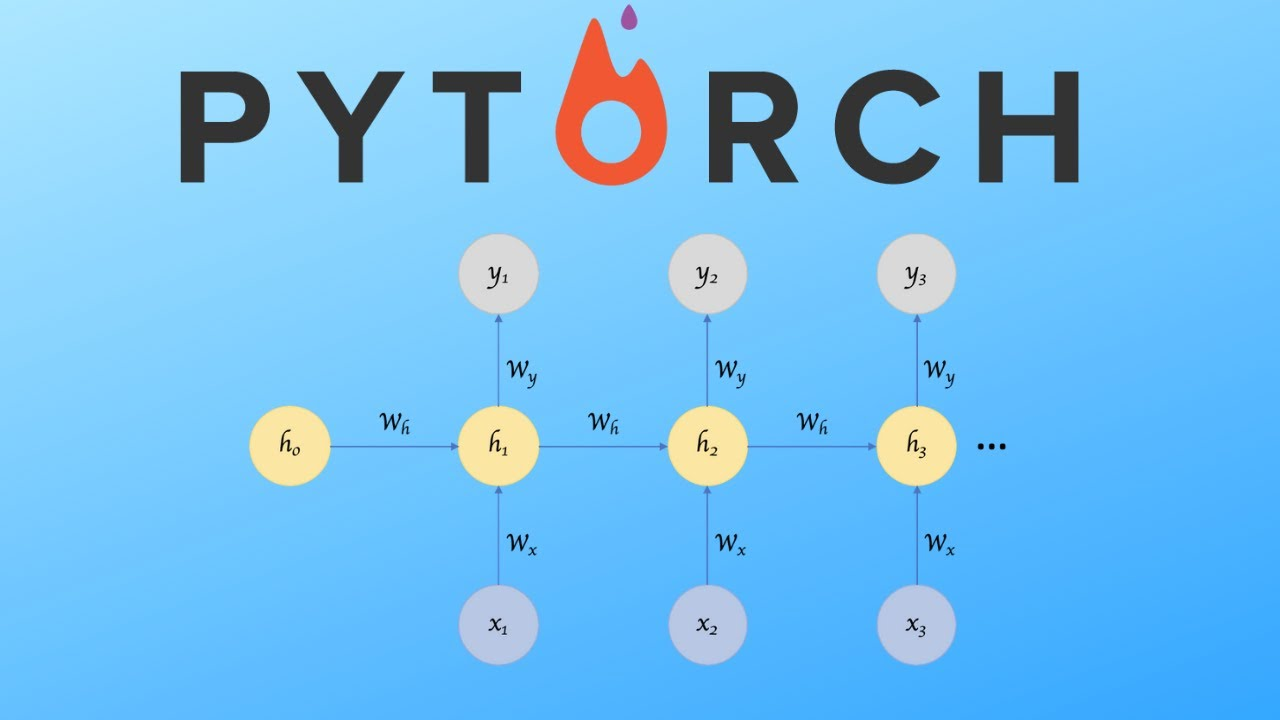1677668905

## TS-mockito

Mocking library for TypeScript inspired by http://mockito.org/

## 1.x to 2.x migration guide

1.x to 2.x migration guide

## Main features

• Strongly typed
• IDE autocomplete
• Mock creation (`mock`) (also abstract classes) #example
• Spying on real objects (`spy`) #example
• Changing mock behavior (`when`) via:
• `thenReturn` - return value #example
• `thenThrow` - throw an error #example
• `thenCall` - call custom method #example
• `thenResolve` - resolve promise #example
• `thenReject` - rejects promise #example
• Checking if methods were called with given arguments (`verify`)
• `anything`, `notNull`, `anyString`, `anyOfClass` etc. - for more flexible comparision
• `once`, `twice`, `times`, `atLeast` etc. - allows call count verification #example
• `calledBefore`, `calledAfter` - allows call order verification #example
• Resetting mock (`reset`, `resetCalls`) #example, #example
• Capturing arguments passed to method (`capture`) #example
• Recording multiple behaviors #example
• Readable error messages (ex. `'Expected "convertNumberToString(strictEqual(3))" to be called 2 time(s). But has been called 1 time(s).'`)

## Installation

`npm install ts-mockito --save-dev`

## Usage

### Basics

``````// Creating mock
let mockedFoo:Foo = mock(Foo);

// Getting instance from mock
let foo:Foo = instance(mockedFoo);

// Using instance in source code
foo.getBar(3);
foo.getBar(5);

verify(mockedFoo.getBar(3)).called();
verify(mockedFoo.getBar(anything())).called();
``````

### Stubbing method calls

``````// Creating mock
let mockedFoo:Foo = mock(Foo);

// stub method before execution
when(mockedFoo.getBar(3)).thenReturn('three');

// Getting instance
let foo:Foo = instance(mockedFoo);

// prints three
console.log(foo.getBar(3));

// prints null, because "getBar(999)" was not stubbed
console.log(foo.getBar(999));
``````

### Stubbing getter value

``````// Creating mock
let mockedFoo:Foo = mock(Foo);

// stub getter before execution
when(mockedFoo.sampleGetter).thenReturn('three');

// Getting instance
let foo:Foo = instance(mockedFoo);

// prints three
console.log(foo.sampleGetter);
``````

### Stubbing property values that have no getters

Syntax is the same as with getter values.

Please note, that stubbing properties that don't have getters only works if Proxy object is available (ES6).

### Call count verification

``````// Creating mock
let mockedFoo:Foo = mock(Foo);

// Getting instance
let foo:Foo = instance(mockedFoo);

// Some calls
foo.getBar(1);
foo.getBar(2);
foo.getBar(2);
foo.getBar(3);

// Call count verification
verify(mockedFoo.getBar(1)).once();               // was called with arg === 1 only once
verify(mockedFoo.getBar(2)).twice();              // was called with arg === 2 exactly two times
verify(mockedFoo.getBar(between(2, 3))).thrice(); // was called with arg between 2-3 exactly three times
verify(mockedFoo.getBar(anyNumber()).times(4);    // was called with any number arg exactly four times
verify(mockedFoo.getBar(2)).atLeast(2);           // was called with arg === 2 min two times
verify(mockedFoo.getBar(anything())).atMost(4);   // was called with any argument max four times
verify(mockedFoo.getBar(4)).never();              // was never called with arg === 4
``````

### Call order verification

``````// Creating mock
let mockedFoo:Foo = mock(Foo);
let mockedBar:Bar = mock(Bar);

// Getting instance
let foo:Foo = instance(mockedFoo);
let bar:Bar = instance(mockedBar);

// Some calls
foo.getBar(1);
bar.getFoo(2);

// Call order verification
verify(mockedFoo.getBar(1)).calledBefore(mockedBar.getFoo(2));    // foo.getBar(1) has been called before bar.getFoo(2)
verify(mockedBar.getFoo(2)).calledAfter(mockedFoo.getBar(1));    // bar.getFoo(2) has been called before foo.getBar(1)
verify(mockedFoo.getBar(1)).calledBefore(mockedBar.getFoo(999999));    // throws error (mockedBar.getFoo(999999) has never been called)
``````

### Throwing errors

``````let mockedFoo:Foo = mock(Foo);

when(mockedFoo.getBar(10)).thenThrow(new Error('fatal error'));

let foo:Foo = instance(mockedFoo);
try {
foo.getBar(10);
} catch (error:Error) {
console.log(error.message); // 'fatal error'
}
``````

### Custom function

You can also stub method with your own implementation

``````let mockedFoo:Foo = mock(Foo);
let foo:Foo = instance(mockedFoo);

when(mockedFoo.sumTwoNumbers(anyNumber(), anyNumber())).thenCall((arg1:number, arg2:number) => {
return arg1 * arg2;
});

// prints '50' because we've changed sum method implementation to multiply!
console.log(foo.sumTwoNumbers(5, 10));
``````

### Resolving / rejecting promises

You can also stub method to resolve / reject promise

``````let mockedFoo:Foo = mock(Foo);

when(mockedFoo.fetchData("a")).thenResolve({id: "a", value: "Hello world"});
when(mockedFoo.fetchData("b")).thenReject(new Error("b does not exist"));
``````

### Resetting mock calls

You can reset just mock call counter

``````// Creating mock
let mockedFoo:Foo = mock(Foo);

// Getting instance
let foo:Foo = instance(mockedFoo);

// Some calls
foo.getBar(1);
foo.getBar(1);
verify(mockedFoo.getBar(1)).twice();      // getBar with arg "1" has been called twice

// Reset mock
resetCalls(mockedFoo);

// Call count verification
verify(mockedFoo.getBar(1)).never();      // has never been called after reset
``````

You can also reset calls of multiple mocks at once `resetCalls(firstMock, secondMock, thirdMock)`

### Resetting mock

Or reset mock call counter with all stubs

``````// Creating mock
let mockedFoo:Foo = mock(Foo);
when(mockedFoo.getBar(1)).thenReturn("one").

// Getting instance
let foo:Foo = instance(mockedFoo);

// Some calls
console.log(foo.getBar(1));               // "one" - as defined in stub
console.log(foo.getBar(1));               // "one" - as defined in stub
verify(mockedFoo.getBar(1)).twice();      // getBar with arg "1" has been called twice

// Reset mock
reset(mockedFoo);

// Call count verification
verify(mockedFoo.getBar(1)).never();      // has never been called after reset
console.log(foo.getBar(1));               // null - previously added stub has been removed
``````

You can also reset multiple mocks at once `reset(firstMock, secondMock, thirdMock)`

### Capturing method arguments

``````let mockedFoo:Foo = mock(Foo);
let foo:Foo = instance(mockedFoo);

// Call method
foo.sumTwoNumbers(1, 2);

// Check first arg captor values
const [firstArg, secondArg] = capture(mockedFoo.sumTwoNumbers).last();
console.log(firstArg);    // prints 1
console.log(secondArg);    // prints 2
``````

You can also get other calls using `first()`, `second()`, `byCallIndex(3)` and more...

### Recording multiple behaviors

You can set multiple returning values for same matching values

``````const mockedFoo:Foo = mock(Foo);

when(mockedFoo.getBar(anyNumber())).thenReturn('one').thenReturn('two').thenReturn('three');

const foo:Foo = instance(mockedFoo);

console.log(foo.getBar(1));    // one
console.log(foo.getBar(1));    // two
console.log(foo.getBar(1));    // three
console.log(foo.getBar(1));    // three - last defined behavior will be repeated infinitely
``````

Another example with specific values

``````let mockedFoo:Foo = mock(Foo);

when(mockedFoo.getBar(1)).thenReturn('one').thenReturn('another one');
when(mockedFoo.getBar(2)).thenReturn('two');

let foo:Foo = instance(mockedFoo);

console.log(foo.getBar(1));    // one
console.log(foo.getBar(2));    // two
console.log(foo.getBar(1));    // another one
console.log(foo.getBar(1));    // another one - this is last defined behavior for arg '1' so it will be repeated
console.log(foo.getBar(2));    // two
console.log(foo.getBar(2));    // two - this is last defined behavior for arg '2' so it will be repeated
``````

Short notation:

``````const mockedFoo:Foo = mock(Foo);

// You can specify return values as multiple thenReturn args
when(mockedFoo.getBar(anyNumber())).thenReturn('one', 'two', 'three');

const foo:Foo = instance(mockedFoo);

console.log(foo.getBar(1));    // one
console.log(foo.getBar(1));    // two
console.log(foo.getBar(1));    // three
console.log(foo.getBar(1));    // three - last defined behavior will be repeated infinity
``````

Possible errors:

``````const mockedFoo:Foo = mock(Foo);

// When multiple matchers, matches same result:
when(mockedFoo.getBar(anyNumber())).thenReturn('one');
when(mockedFoo.getBar(3)).thenReturn('one');

const foo:Foo = instance(mockedFoo);
foo.getBar(3); // MultipleMatchersMatchSameStubError will be thrown, two matchers match same method call
``````

### Mocking interfaces

You can mock interfaces too, just instead of passing type to `mock` function, set `mock` function generic type Mocking interfaces requires `Proxy` implementation

``````let mockedFoo:Foo = mock<FooInterface>(); // instead of mock(FooInterface)
const foo: SampleGeneric<FooInterface> = instance(mockedFoo);
``````

### Mocking types

You can mock abstract classes

``````const mockedFoo: SampleAbstractClass = mock(SampleAbstractClass);
const foo: SampleAbstractClass = instance(mockedFoo);
``````

You can also mock generic classes, but note that generic type is just needed by mock type definition

``````const mockedFoo: SampleGeneric<SampleInterface> = mock(SampleGeneric);
const foo: SampleGeneric<SampleInterface> = instance(mockedFoo);
``````

### Spying on real objects

You can partially mock an existing instance:

``````const foo: Foo = new Foo();
const spiedFoo = spy(foo);

when(spiedFoo.getBar(3)).thenReturn('one');

console.log(foo.getBar(3)); // 'one'
console.log(foo.getBaz()); // call to a real method
``````

You can spy on plain objects too:

``````const foo = { bar: () => 42 };
const spiedFoo = spy(foo);

foo.bar();

console.log(capture(spiedFoo.bar).last()); // 
``````

### Thanks

Author: NagRock
Source Code: https://github.com/NagRock/ts-mockito1594460183

## Pytorch RNN Example (Recurrent Neural Network)

In this video we go through how to code a simple rnn, gru and lstm example. Focus is on the architecture itself rather than the data etc. and we use the simple MNIST dataset for this example.

#pytorch #rnn #neural network1596825840

## A Comparative Analysis of Recurrent Neural Networks

Recurrent neural networks, also known as RNNs, are a class of neural networks that allow previous outputs to be used as inputs while having hidden states. RNN models are mostly used in the fields of natural language processing and speech recognition.

The vanishing and exploding gradient phenomena are often encountered in the context of RNNs. The reason why they happen is that it is difficult to capture long term dependencies because of multiplicative gradient that can be exponentially decreasing/increasing with respect to the number of layers.

Gated Recurrent Unit (GRU) and Long Short-Term Memory units (LSTM) deal with the vanishing gradient problem encountered by traditional RNNs, with LSTM being a generalization of GRU.

1D Convolution_ layer_ creates a convolution kernel that is convolved with the layer input over a single spatial (or temporal) dimension to produce a tensor of outputs. It is very effective for deriving features from a fixed-length segment of the overall dataset. A 1D CNN works well for natural language processing (NLP).

## DATASET: IMDb Movie Review

TensorFlow Datasets is a collection of datasets ready to use, with TensorFlow or other Python ML frameworks, such as Jax. All datasets are exposed as `[_tf.data.Datasets_](https://www.tensorflow.org/api_docs/python/tf/data/Dataset)`, enabling easy-to-use and high-performance input pipelines.

“imdb_reviews”

This is a dataset for binary sentiment classification containing substantially more data than previous benchmark datasets. It provides a set of 25,000 highly polar movie reviews for training, and 25,000 for testing.

## Import Libraries

``````import pandas as pd
import numpy as np
import matplotlib.pyplot as plt
import seaborn as sns
%matplotlib inline
``````

``````import tensorflow as tf
import tensorflow_datasets

imdb
``````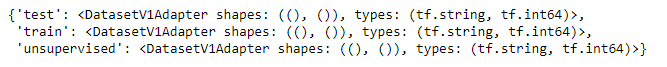``````info
``````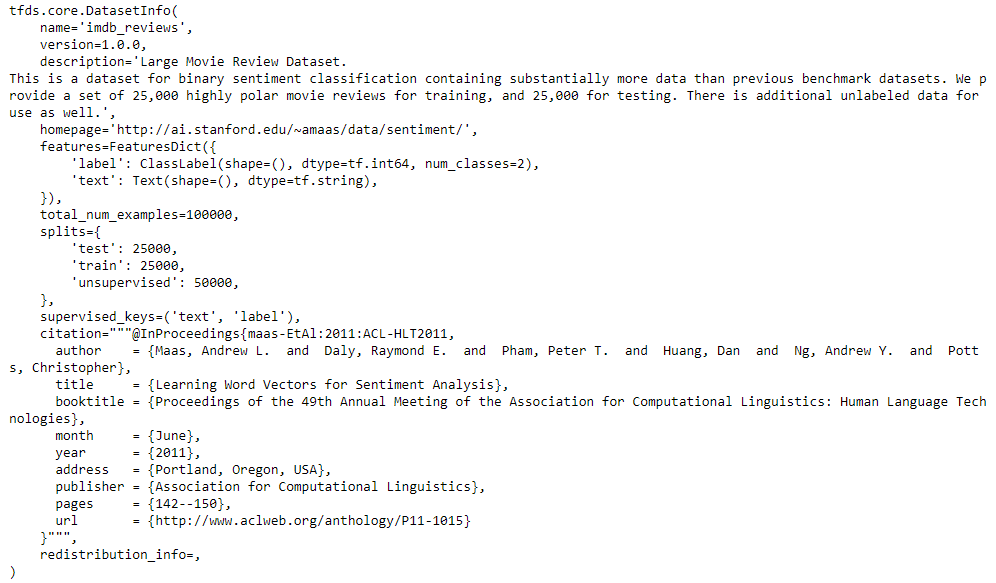## Training and Testing Data

``````train_data, test_data=imdb['train'], imdb['test']

training_sentences=[]
training_label=[]
testing_sentences=[]
testing_label=[]
for s,l in train_data:
training_sentences.append(str(s.numpy()))
training_label.append(l.numpy())
for s,l in test_data:
testing_sentences.append(str(s.numpy()))
testing_label.append(l.numpy())
training_label_final=np.array(training_label)
testing_label_final=np.array(testing_label)
``````

``````vocab_size=10000
embedding_dim=16
max_length=120
trunc_type='post'
oov_tok='<oov>'
from tensorflow.keras.preprocessing.text import Tokenizer
tokenizer= Tokenizer(num_words=vocab_size, oov_token=oov_tok)
tokenizer.fit_on_texts(training_sentences)
word_index=tokenizer.word_index
sequences=tokenizer.texts_to_sequences(training_sentences)
testing_sequences=tokenizer.texts_to_sequences(testing_sentences)
from tensorflow.keras.models import Sequential
from tensorflow.keras.layers import Dense, Dropout, Embedding
``````

## Multi-layer Bidirectional LSTM

#imdb #convolutional-network #long-short-term-memory #recurrent-neural-network #gated-recurrent-unit #neural networks1594524540

## The Recurrent Neural Network (RNNs)

A recurrent neural network (RNN) is an input node (hidden layer) that feeds sigmoid activation. The way an RNN does this is to take the output of one neuron and return it as input to another neuron or feed the input of the current time step to the output of earlier time steps. Here you feed the input from the previous times step by step into the input of the current times and vice versa.This can be used in a variety of ways, such as through learning gates with known variations or a combination of sigmoid activation and a number of other types of neural networks.

Some of the applications for RNNs include predicting energy demand, predicting stock prices, and predicting human behavior. RNNs are modeled over time — based and sequence-based data, but they are also useful in a variety of other applications.

A recurrent neural network is an artificial neural network used for deep learning, machine learning, and other forms of artificial intelligence (AI). They have a number of attributes that make them useful for tasks where data needs to be processed sequentially.

To get a little more technical, recurring neural networks are designed to learn a sequence of data by traversing a hidden state from one step of the sequence to the next, combined with the input, and routing it back and forth between the inputs. RNN are neural networks that are designed for the effective handling of sequential data but are also useful for non-sequential data.

These types of data include text documents that can be seen as a sequence of words or audio files in which you can see a sequence of sound frequencies and times. The more information about the output layer is available, the faster it can be read and sequenced, and the better its performance.

#recurrent-neural-network #lstm #rnn #artificial-intelligence #neural network1594366200

## Recurrent Neural Networks for Multilabel Text Classification Tasks

The purpose of this project is to build and evaluate Recurrent Neural Networks(RNNs) for sentence-level classification tasks. I evaluate three architectures: a two-layer Long Short-Term Memory Network(LSTM), a two-layer Bidirectional Long Short-Term Memory Network(BiLSTM), and a two-layer BiLSTM with a word-level attention layer. Although they do learn useful vector representation, BiLSTM with attention mechanism focuses on necessary tokens when learning text representation. To that end, I’m using the 2019 Google Jigsaw published dataset on Kaggle labeled “Jigsaw Unintended Bias in Toxicity Classification.” The dataset includes 1,804,874 user comments, with the toxicity level being between 0 and 1. The final models can be used for filtering online posts and comments, social media policing, and user education.

## Recurrent Neural Networks Overview

RNNs are neural networks used for problems that require sequential data processing. For instance:

• In a sentiment analysis task, a text’s sentiment can be inferred from a sequence of words or characters.
• In a stock prediction task, current stock prices can be inferred from a sequence of past stock prices.

At each time step of the input sequence, RNNs compute the output yt and an internal state update ht using the input xt and the previous hidden-state ht-1. They then pass information about the current time step of the network to the next. The hidden-state ht summarizes the task-relevant aspect of the past sequence of the input up to t, allowing for information to persist over time.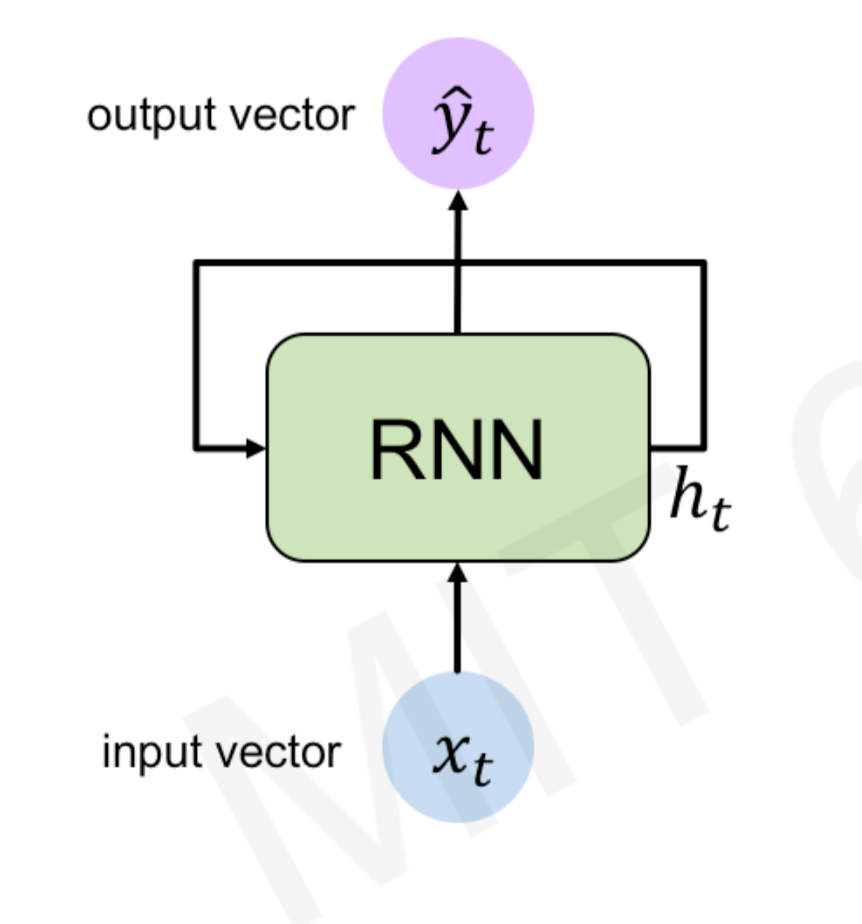Recurrent Neural Network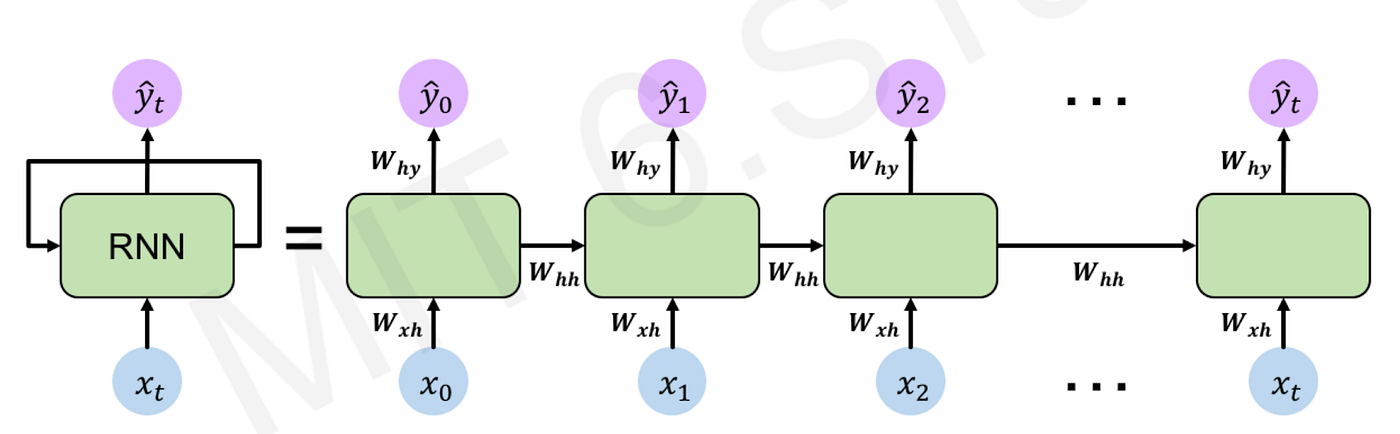Recurrent Neural Network

During training, RNNs re-use the same weight matrices at each time step. Parameter sharing enables the network to generalize to different sequence lengths. The total loss is a sum of all losses at each time step, the gradients with respect to the weights are the sum of the gradients at each time step, and the parameters are updated to minimize the loss function.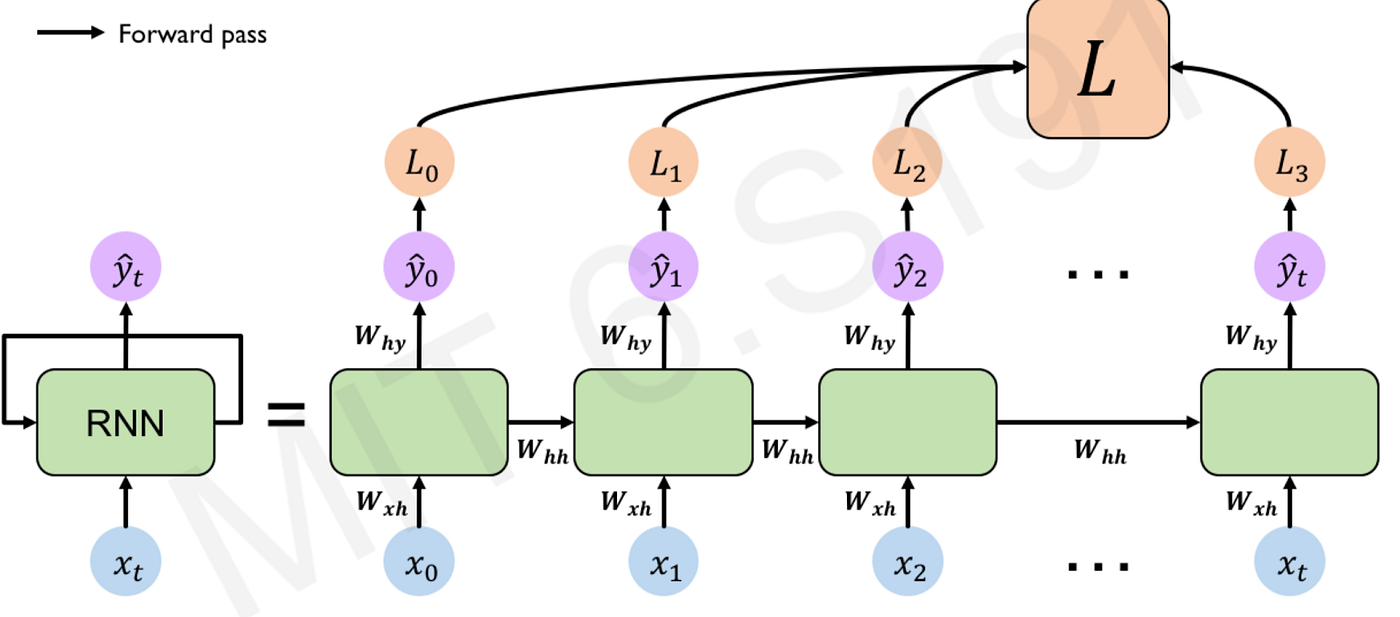forward pass: compute the loss function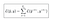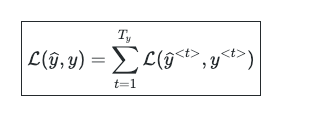loss function